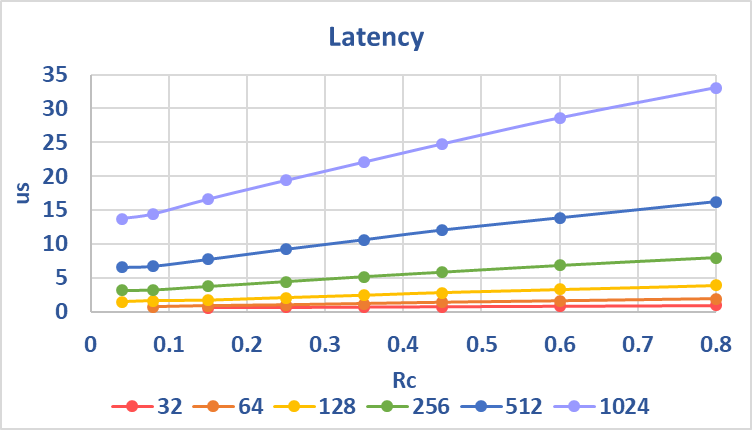ID 683766
Date 9/30/2020
Public

## 4.2. 5G Polar IP Throughput and Latency

Throughput and latency can vary for different input frames, different input LLR values, different locations of frozen bits and parity check bits, and the number of frozen bits that determines code rate. The latency improves by about 5% when you turn off CRC and interleaving, but the throughput remains the same. The NUM_LIST = 8 decoder has approximately 10% longer latency and lower throughput compared to the NUM_LIST = 4 decoder.
Figure 21. Encoder latency and throughputClock frequency = 370MHz. CRC and interleaving off.Figure 22. Encoder latency and throughputCRC = 6 and interleaving onFor the decoder figures, Rc is the code rate, which equals to number_of_information_bits (K) / code_block_length (N).

Figure 23. Decoder latencyClock frequency = 370MHz. NUM_LIST = 4Figure 24. Decoder throughput (codeword bit)Clock frequency = 370MHz. NUM_LIST = 4Figure 25. Decoder throughput (information bit)Clock frequency = 370MHz. NUM_LIST = 4